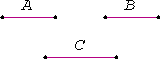# Proposition 9

Magnitudes which have the same ratio to the same equal one another; and magnitudes to which the same has the same ratio are equal.

Let each of the magnitudes A and B have the same ratio to C.

I say that A equals B.V.8

Otherwise, each of the magnitudes A and B would not have the same ratio to C, but they do, therefore A equals B.

Next, let C have the same ratio to each of the magnitudes A and B.

I say that A equals B.

V.8

Otherwise, C would not have the same ratio to each of the magnitudes A and B, but it does, therefore A equals B.

Therefore, magnitudes which have the same ratio to the same equal one another; and magnitudes to which the same has the same ratio are equal.

Q.E.D.

## Guide

This converse to proposition V.7 has two statements:

If a : c = b : c, then a = b.

If c : a = c : b, then a = b.

Besides the previous proposition, the proof relies on the law of trichotomy for ratios, the part which says that a : b < a : c and a : b = a : c cannot both occur. Although Euclid didn’t prove that, it follows easily from the definitions in V.Def.5 and V.Def.7.

This proposition relies on using V.Def.4 as an axiom of comparability through its use of the previous proposition. The axiom is required since the statement of the proposition is false when a is c + y, and b is c + 2y where y is an infinitesimal with respect to c.

This proposition is used occasionally in Books VI, VII, X, XI, and XII to conclude equality of geometric magnitudes.